• Call Now

1800-102-2727•

# Amplitude Modulation

A news channel wants to broadcast news live on its prime show. Then how do they do it, and how will they deliver important information to the people? Yes, they need a good quality communication system which transmits the information in the form of signals. But the raw signals may be very weak in quality or there might be interference from other signals, hence we need a device that will improve the quality of transmission signal.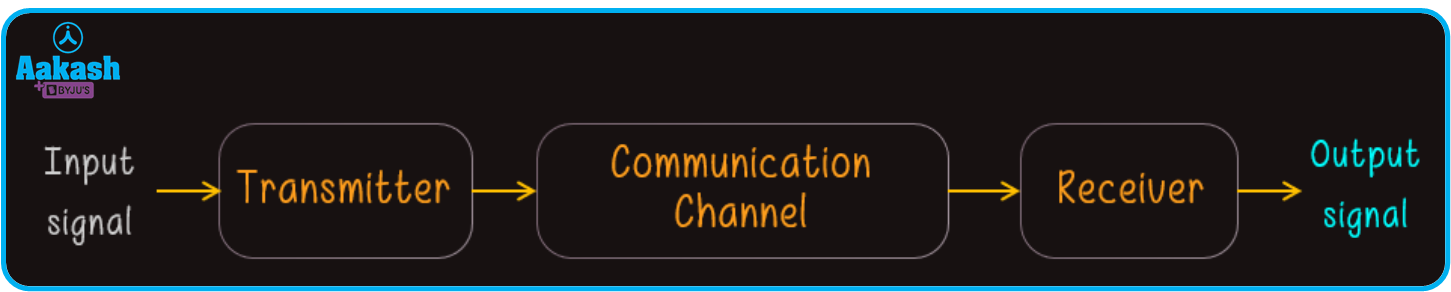Parts of the communication system

Table of content

• What is Modulation?
• Types of modulation
• What is Amplitude Modulation?
• Modulation Index
• Derivation for amplitude modulated wave
• Frequency spectrum and Bandwidth of AM wave
• Uses of Amplitude modulation
• Practice problem
• FAQs

## What is modulation?

At the transmitter, the information contained in the message signal is of low frequency and therefore is superimposed on a high-frequency wave which acts as a carrier of the information. The whole process is known as modulation and it is done by a device called modulator.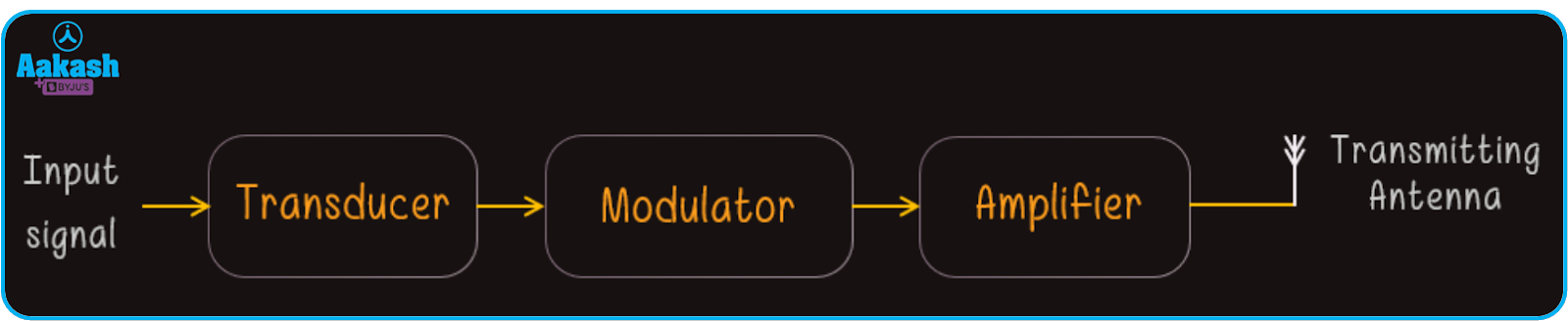## Types of modulation

Modulation is divided into two parts based on the type of carrier wave.

1. Continuous wave modulation
1. Amplitude modulation
2. Frequency modulation
3. Phase modulation
1. Pulse wave modulation
1. Pulse amplitude modulation
2. Pulse width modulation
3. Pulse position modulation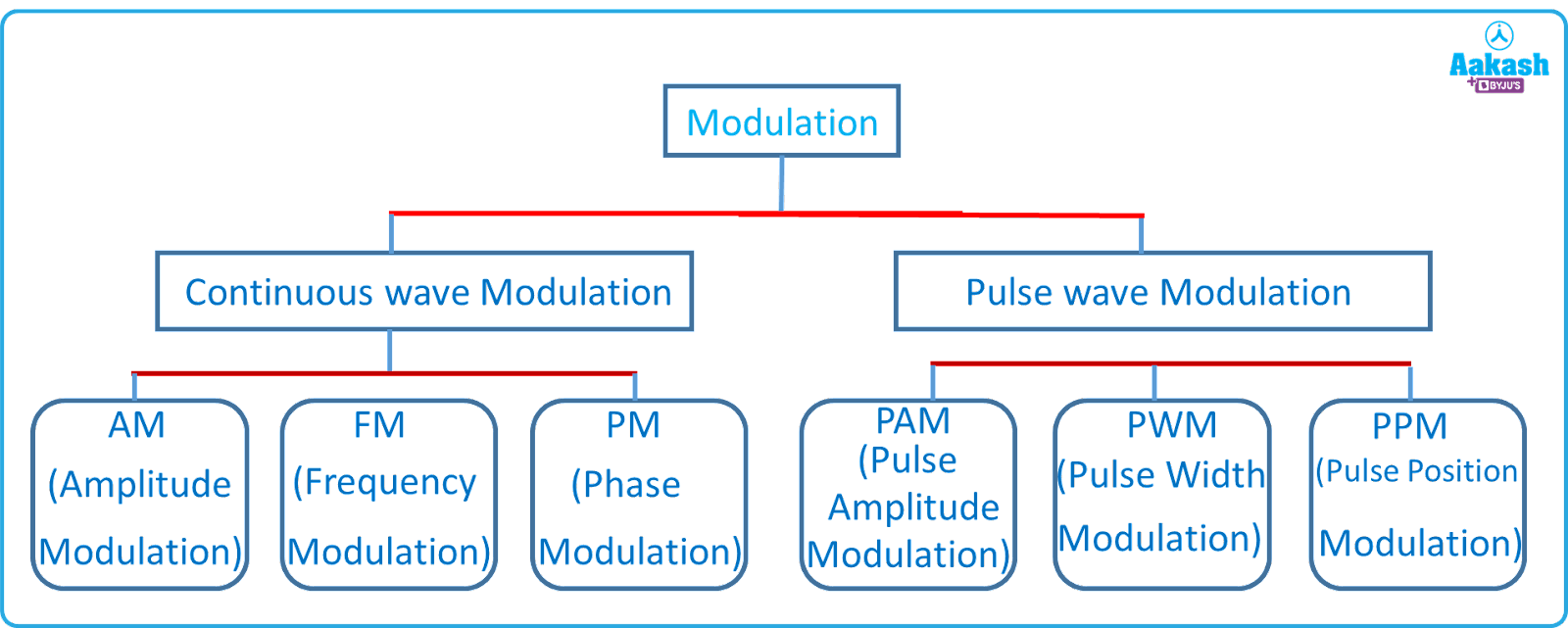## What is Amplitude modulation?

If the amplitude of the carrier wave signal is varied in accordance with the amplitude of the message signal, then the modulation is called amplitude modulation. Here the frequency and phase of the carrier and transmitted signal are kept constant.

Since, the frequency of message signal is very low (20 Hz-20 kHz), a high frequency carrier signal is required to transmit the message signal till it reaches the receiver. The waveform of the message signal and carrier signal is depicted below.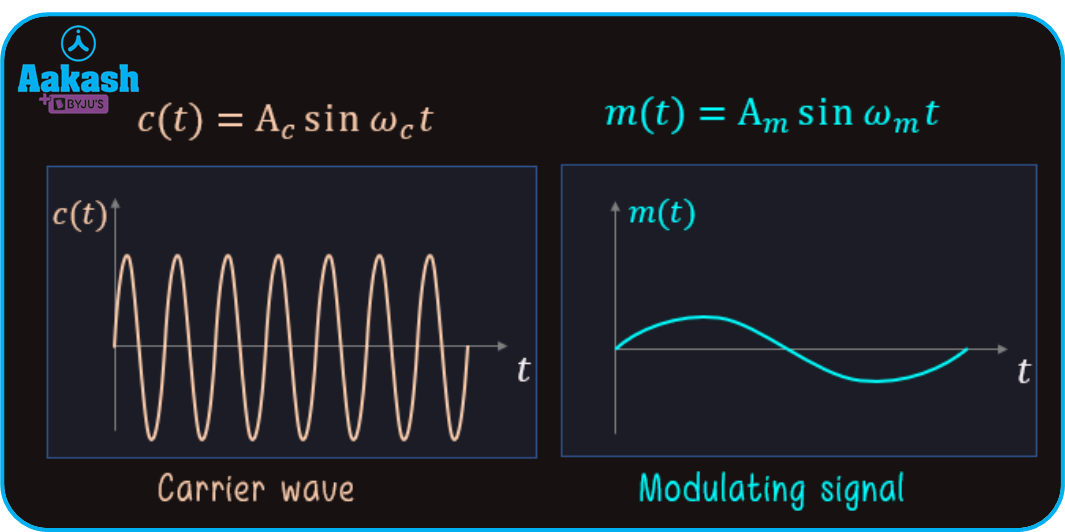Waveform of carrier signal and message signal

During the amplitude modulation process, the message signal is superimposed on a high-frequency carrier signal in such a way that the amplitude of the carrier signal is greater than the amplitude of the message signal while constant frequency and phase is maintained between the carrier and transmitted signal. The superposition of these two signals will form a modulated wave known as amplitude modulated wave.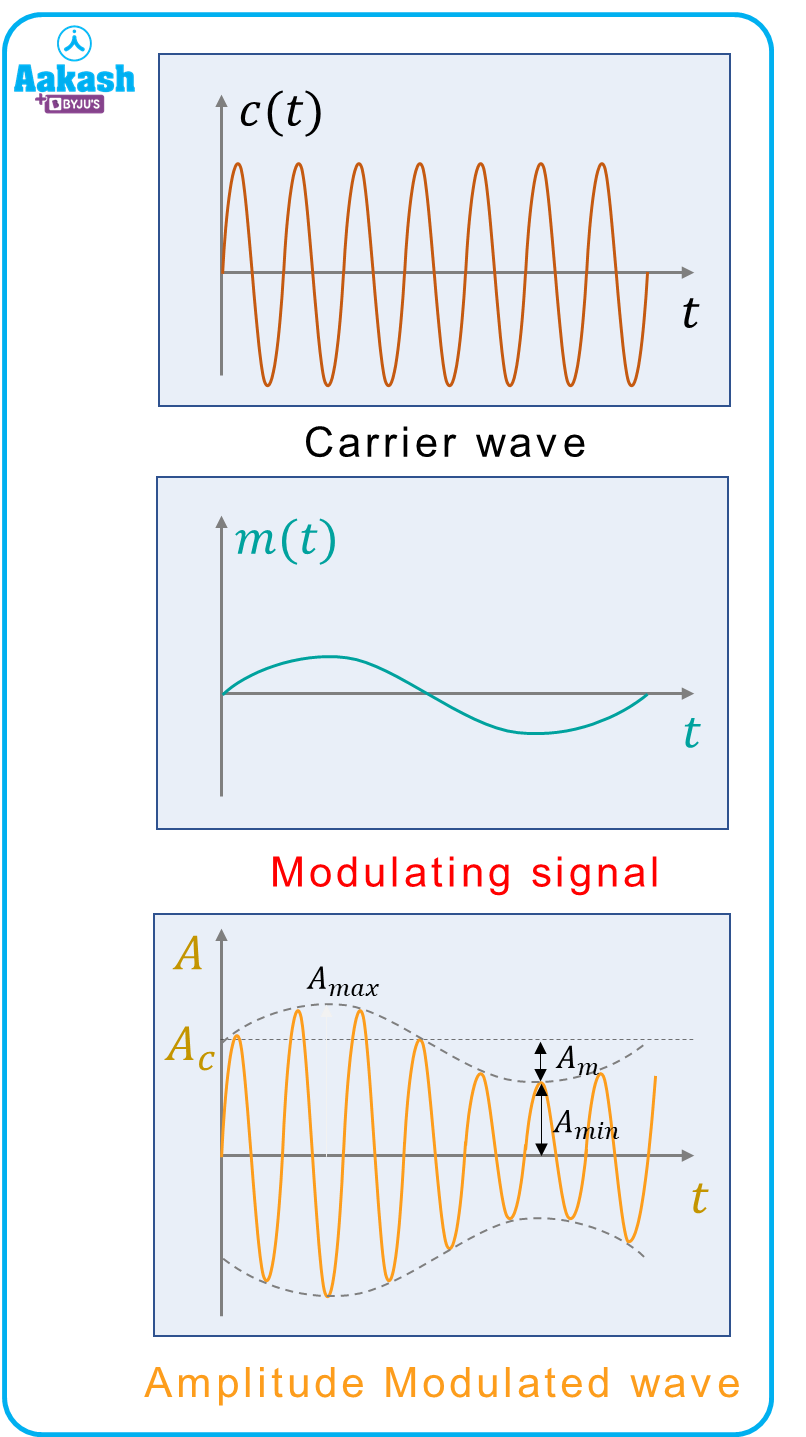Waveform of amplitude modulated wave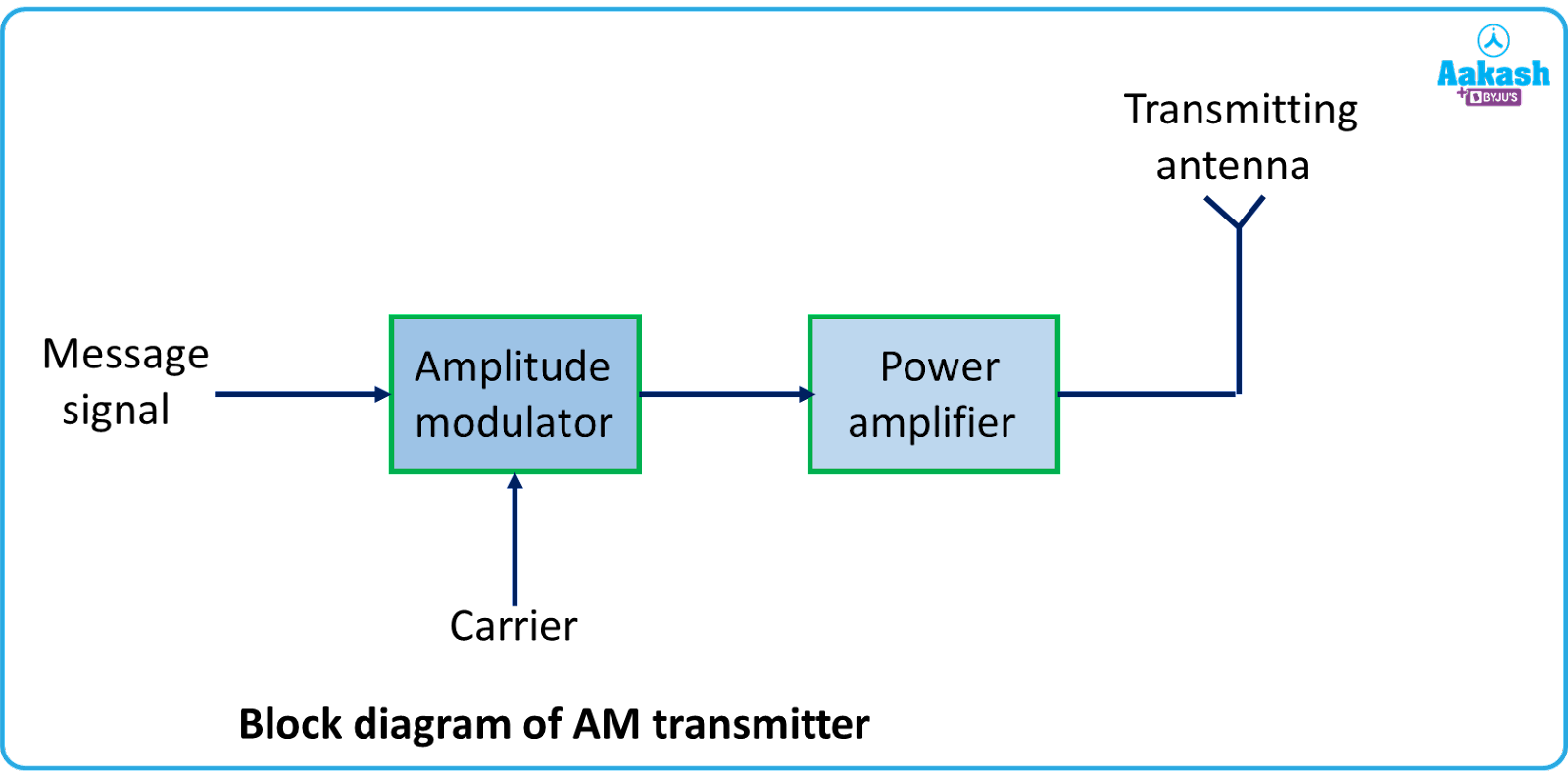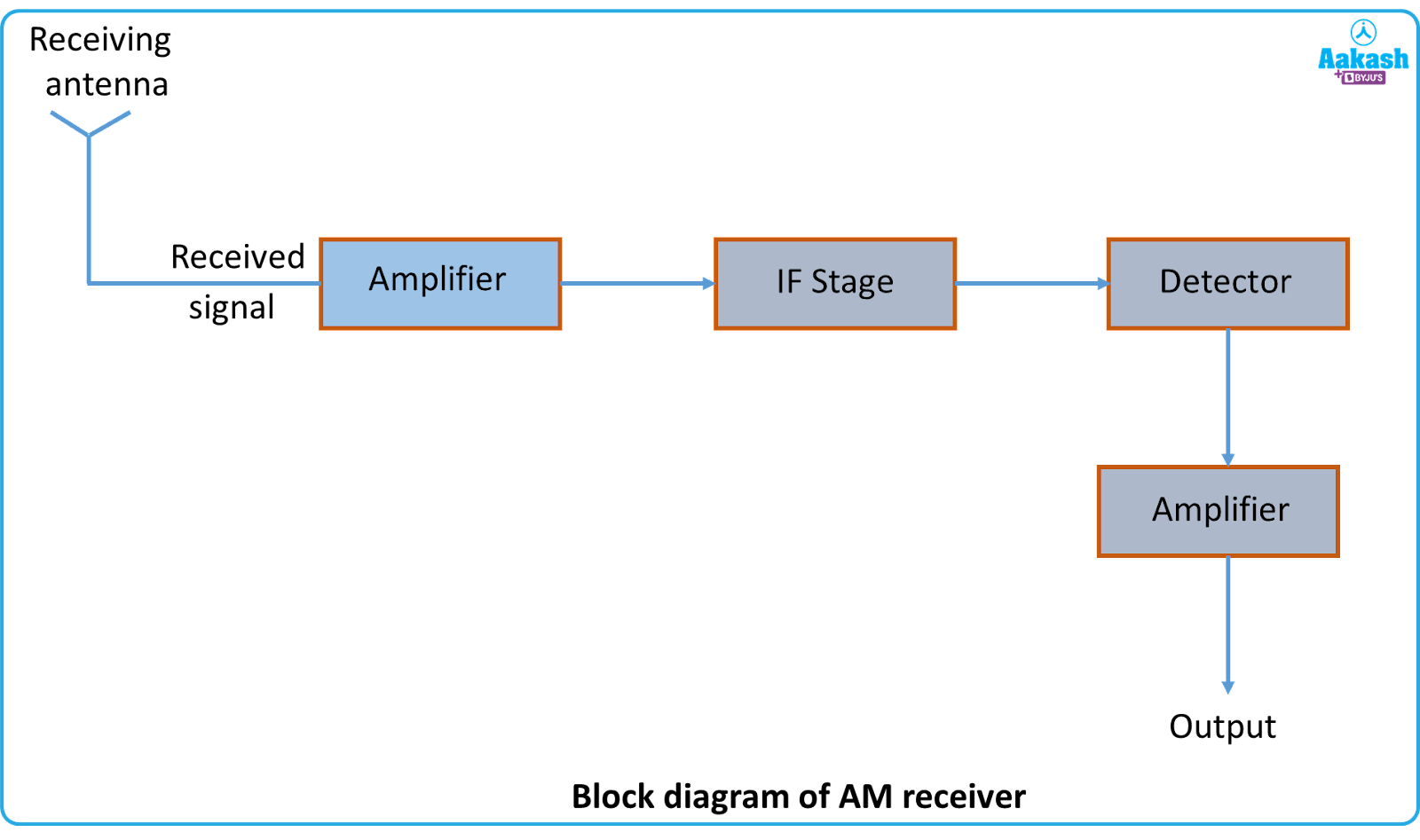## Modulation Index ()

Modulation index is the ratio of amplitude of a message signal to the amplitude of a carrier signal. Mathematically it is written as,

Modulation index determines how good the transmitted signal is in quality. As the modulation index is increased, the audio signal on reception becomes better.

Modulation index is kept less than or equal to 1, in order to avoid any distortion.

## Derivation for amplitude modulated wave

Let the carrier wave and message signal be represented as,

$c\left(t\right)={A}_{c}sin{\omega }_{c}tandm\left(t\right)={A}_{m}sin{\omega }_{m}t$

The waveform of respective signals is shown in the below figure,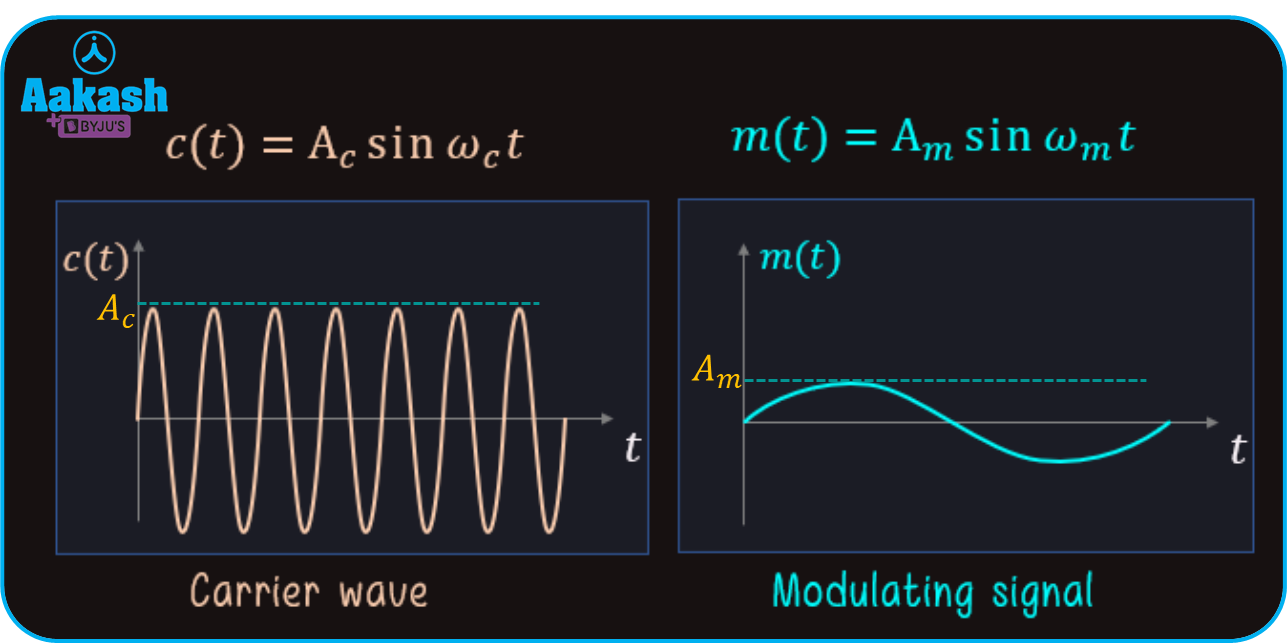Waveform of carrier signal and message signal

Now after superposition of these two signals, the modulated signal is given by,

Here the frequency of the AM wave is equal to the frequency of the carrier signal.

The Waveform of the modulated signal is depicted below,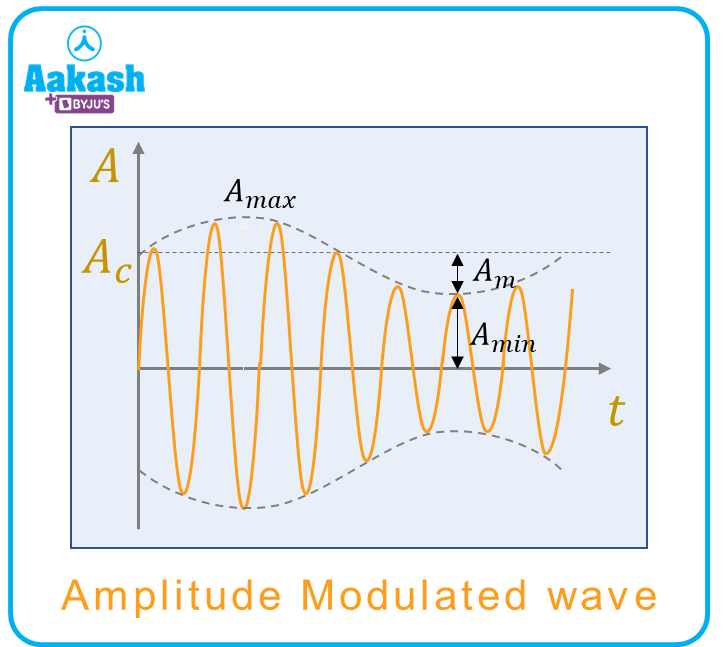Where, ${A}_{max}={A}_{c}+{A}_{m}and{A}_{min}={A}_{c}-{A}_{m}$

Therefore,

Now from equation (i),

Where, $\frac{{A}_{m}}{{A}_{c}}=\mu =$ modulation index

Then from equation (ii),

${c}_{m}\left(t\right)=\left(1+\mu sin{\omega }_{m}t\right){A}_{c}sin{\omega }_{c}t$

$⇒{c}_{m}\left(t\right)=\left({A}_{c}sin{\omega }_{c}t+\mu {A}_{c}sin{\omega }_{c}tsin{\omega }_{m}t\right)$

Now from trigonometric relation,

$sinAsinB=\frac{1}{2}\left[cos\left(A-B\right)-cos\left(A+B\right)\right]$

Then,

Equation (iii) represents the wave equation of the AM wave.

Frequency spectrum and Bandwidth of AM wave

The wave equation of Amplitude Modulated wave is given by,

${c}_{m}\left(t\right)={A}_{c}sin{\omega }_{c}t+\frac{\mu {A}_{c}}{2}\left[cos\left({\omega }_{c}-{\omega }_{m}\right)-cos\left({\omega }_{c}+{\omega }_{m}\right)\right]$

In the above equation, (c-m) and (c+m) are known as Lower Side frequency and Upper Side frequency respectively.

The plot between amplitude (A) and for an AM wave is given below,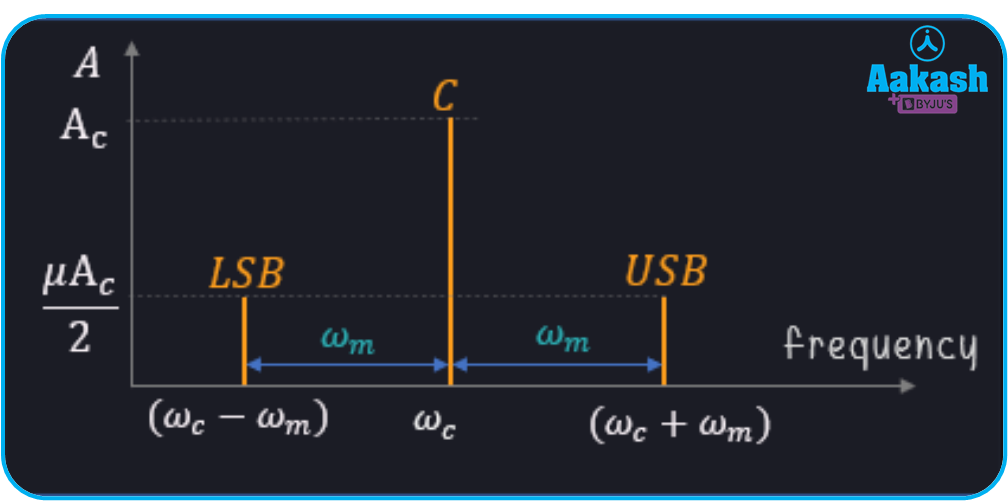Plot between amplitude (A) and for an AM wave

As we can see from the above figure, the modulated signal consists of two frequencies along with the carrier frequency c which are known as Side Bands.

If (fc-fm) and (fc+fm) are the frequency corresponding to (c-m) and (c+m)

Then, (fc-fm) frequency of Lower Side Band

And (fc+fm) frequency of Upper Side Band

The Bandwidth of the AM wave is given by,

Bandwidth $={f}_{USB}-{f}_{LSB}=\left({f}_{c}+{f}_{m}\right)-\left({f}_{c}-{f}_{m}\right)=2{f}_{m}$

 Advantages Disadvantages Easier to implement. Power consumption is high. Circuits of transmitter and receiver are simpler. Bandwidth is very high compared to information signals which may cause poor audio quality. The area at the reception is high as compared to other modulation methods. It can not distinguish between voice and noise i.e., low frequency disturbances.

## Uses of Amplitude modulation

• It is used in the broadcasting industry for short, medium as well as long wavebands.
• Due to its very high-frequency transmission ability, it is used in many air-borne applications like ground-to-air radio communications.
• It is also used in short-range wireless applications such as Wi-Fi to cellular telecommunications.

## Practice problems

Q. A message signal of 10 kHz frequency is to be transmitted to a receiver. The amplitude of peak voltage is 20 V which is used to modulate the carrier signal frequency of 2 MHz and voltage of 40 V. Determine the modulation index.

Ans: The peak voltage of the modulating signal, Am=20 V

And the peak voltage of the carrier signal, Ac=40 V

Then the modulation index is given by,

$\mu =\frac{20}{40}=0.5$

Q. The peak voltages of a message wave and a carrier wave is 30 V and 50 V respectively. Find out the maximum and minimum voltages of the respective modulated wave.

Ans: Given, the peak voltage of the message signal, Am=30 V

And the peak voltage of the message signal, Ac=50 V

The maximum and minimum amplitudes of voltage corresponding to the modulated wave is,

Amax=Ac+Am

and Amin=Ac-Am

Then the maximum and minimum amplitudes will be,

Amax=50+30=80 V

Amin=50-30=20 V

Q. A carrier frequency of 20 GHz is the working condition of a telephonic company but only 20% is used for transmission. Then find the number of telephonic channels which can be transmitted simultaneously if 10 kHz bandwidth is required for each channel.

Ans: The carrier frequency of carrier signal is 20 GHz.

If only 20% of the strength is utilised, then

Useful carrier frequency =20 GHz 0.2=4 GHz

Hence, only 4 GHz of frequency is being used for the transmission.

Now the bandwidth for each channel is given as 10 kHz.

Then the number of channels that can be transmitted are,

Hence, 4 105 number of channels can be transmitted simultaneously if 10 kHz bandwidth is required for each channel.

4. Find the lower sideband, upper sideband and the bandwidth of AM transmission if the carrier frequency is 50 kHz and the message signal frequency is 5 kHz.

Ans: Given, Carrier wave frequency, fc=50 kHz

And message wave frequency, fm=5 kHz

Now the lower sideband and upper sideband is given by,

Then the Bandwidth of AM transmission is,

This can also be calculated as,

## FAQs

Q. What is amplitude modulation?
Ans.
The modulation in which the amplitude of the carrier wave is kept different (generally greater than the message signal to avoid distortion) from the amplitude of the message signal by keeping the frequency and phase of the modulated and the carrier signal constant, is called amplitude modulation.

Q. When does distortion occur in transmission?
Ans.
The distortion in transmission occurs when the modulating signal becomes stronger than the carrier wave signal. Thereby, there may be the chances of passing incorrect information.

Q. Write the total power in an amplitude modulated wave.
Ans.
The total power in amplitude modulated wave is given by,

${P}_{t}={P}_{c}+{P}_{USB}+{P}_{LSB}={P}_{c}+\frac{{\mu }^{2}}{4}{P}_{c}+\frac{{\mu }^{2}}{4}{P}_{c}$

${P}_{t}={P}_{c}\left(1+\frac{{\mu }^{2}}{2}\right)$

Where, Pc= Power in carrier wave

PUSB= Power in upper sideband

PLSB= Power in lower sideband

$\mu =$ Modulation index

Q. What is the wave character of an AM wave?
Ans.
An amplitude modulated wave is transverse in nature since it is the superposition of different amplitude waves (carrier wave and message signal). In amplitude modulation, the amplitude (i.e, in turn the signal strength) of the wave is varied in proportion to that of the message signal. This is done commonly in an audio signal.

Talk to our expert
Resend OTP Timer =
By submitting up, I agree to receive all the Whatsapp communication on my registered number and Aakash terms and conditions and privacy policy